# Catch up on the latest AI articles# Barbershop Generates Composite Images Of Multiple Faces!

3 main points
✔️ Image generation task by combining two face images
✔️ Newly proposed FS space instead of W space used in CycleGAN2 etc.
✔️ Generated images outperform existing methods in all metrics

Written by Peihao Zhu, RameenAbdal, John Femiani, Peter Wonka
(Submitted on 2 Jun 2021)

Subjects: Computer Vision and Pattern Recognition (cs. CV); Graphics (cs.GR)code:.## first of all

Image editing using Generative Adversarial Network (GAN) has recently become widely used in professional applications and social media photo editing tools for general users. In particular, tools for editing human face pictures have attracted much attention. In this paper, we propose a new tool for image editing by generating a composite image that combines elements of multiple images.

Recently, face editing by manipulating latent space has been used successfully, but these images are manipulated by changing global attributes such as pose, expression, gender, and age. The synthesis task we wish to perform in this paper is challenging for a number of reasons. First, the visual properties of each part are not independent of each other. In the case of the hair, it is affected by the ambient light and the transmitted light from the face, clothes, and background. Also, the face and shoulders affect the hair and shadows. For these reasons, if the overall consistency of the image is not taken into account, artifacts will occur where different regions of the image will appear disjointed even if each part is of high quality. Therefore, in this paper, we propose a new $FS$ latent space that allows for coarse spatial location control of features by the structure tensor $F$ and fine control of global style attributes by the appearance code $S$.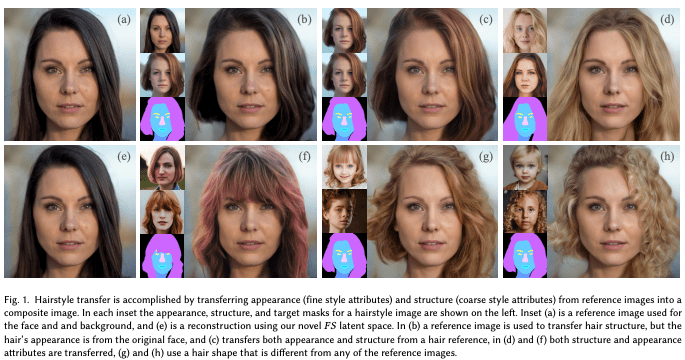The method proposed in this paper is shown in Fig. 1. It can transfer only the shape of the hair in the target image to the original image (b). It is also able to transfer the difference between straight hair and curly hair (d~g).

## technique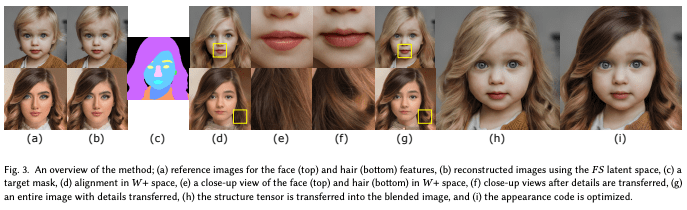The flow of the proposed method is shown in Fig. 3. The general flow is as follows:

1. Segmentation of the referenced image is generated or manually generated
2. The latent code C^{align}_k=(F^{align}_k,S^{align}_k) is detected from each segmented image $Z_k$.
3. For each $k$, copy the region $k$ of F^{align}_k to form the tensor $F^{blend}$ of the joint structure
4. The weights of S^{align}_k are derived so that the appearance code $S^{blend}$ is aligned as an image

### Initial Segmentation

The first step is to segment the reference image. This selects the region that should be copied to the target image. Let $M_k=SEGMENT(Z_k)$ denote the segmentation of the reference image $Z_k$ and $SEGMENT()$ is a segmentation network such as BiSeNET. The goal in this step will be to form a composite image $Z^{blend}$ that matches the target segmentation mask $M$ so that the visual properties of $Z^{blend}$ are transferred from the original reference image $Z_k$ at positions where $M=k$. Here, the target mask $M(x,y)$ for each pixel is set to a value $k$ that satisfies the condition $M_k(x,y)=k$. If multiple $k$ satisfy the condition, the larger $k$ is selected. For example, some parts of the hair are naturally covered by the skin. In this case, both skin and hair labels are applied, but since $k$ is larger for the hair label, the hair label is applied instead of the skin label.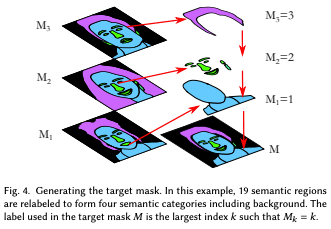### embedding

Before compositing the images, we first align each image with the target mask $M$. This is an important step because, as mentioned in the "Introduction", parts such as eyes and nose are not independent of each other and depend on the pose of the whole head. It consists of "Reconstruction" to find the latent code $C^{rec}_k$ to reconstruct the input image $Z_k$ to align the reference image, and "Alignment" to find the latent code C^{align}_k to minimize the cross-entropy between the generated image and the target mask. Alignment" to find the latent code C^{alignment}_k that minimizes the cross-entropy between the generated image and the target mask.

Reconstruction Reconstruction

Given $Z_k$ as an input image, we find $C^{rec}_k$ such that $G(C^{rec}_k)$ can reconstruct $Z_k$ ($G()$ is a generator). As a method to do so, in this paper we use II2S to derive $W^{rec}_k$ using the W+ space of StyleGAN2 to initialize it. However, W+ space is not sufficient to capture facial details such as wrinkles and moles; one approach is noise embedding, which is almost perfect in terms of reconstruction but leads to overfitting that appears as artifacts in image editing and compositing. A comparison between the W+ and FS spaces is shown in Fig. 5.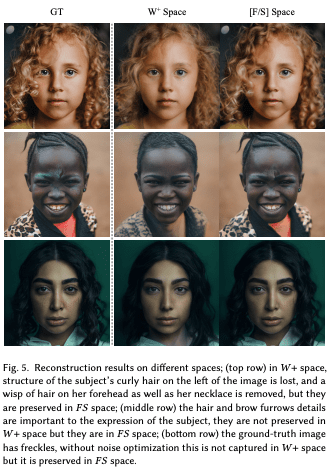Fig. 5 shows that more detailed information is captured in FS space. We use the output of the style block of the generator as a spatially correlated structure tensor $F$ and replace the corresponding block in W+ space. For simplicity, we use style block 8 here. We form $F^{init}_k=G_8(w^{rec}_k)$ as the initial structure tensor and initialize the appearance code $S^{init}_k$ with 10 blocks of $w^{rec}_k$. Then we derive $C^{rec}_k$: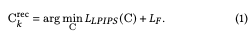Here.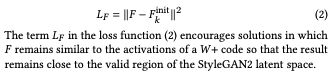・Alignment

Each input image $Z_k$ is encoded by $C^{rec}_k$, which consists of a tensor $F^{rec}_k$ and an appearance code $S^{rec}_k$. Although $C^{rec}_k$ captures the appearance of the image, it is not aligned with the more detailed part, the target segmentation. Therefore, we derive a latent code C^{align}_k that matches the target segmentation and exists near $C^{rec}_k$, which shows the appearance. However, directly optimizing C^{align}_k is difficult because $F^{rec}_k$ is spatially correlated. Therefore, we first transfer the details of $F^{rec}_k$ to F^{align}_k.

Use masked style loss to preserve the style between the aligned image G(w^{alignment}) and the original image $Z_k$.

gram matrix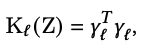where $\gamma$ is the matrix obtained by the activation of layer $l$ of the VGG network. Then we define the mask: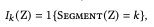Here, $1\{\}$ is an indicator function, so $I_k$ is an indicator of the domain of the semantic category $k$.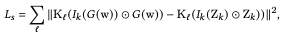The style loss is then indicated by the magnitude of the difference between the gram matrix of the image generated by the latent code $w$ and the target image $Z_k$, and is evaluated only within the semantic domain $k$ of each image:$I_k(Z_k)\bigodot Z_k$ is the formula for masking by setting pixels outside the semantic region $k$ to 0. The cycle loss is as follows: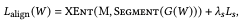where $XEnt()$ is a multiclass cross-entropy function.

The next equation for transferring the structure and appearance from the image $Z_k$ to $F_k$ is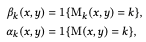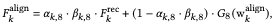If the semantic class of the target image and the reference image is the same, it copies $F^{rec}_k$. If the semantic class of the target image and the reference image is the same, $F^{rec}_k$ is copied as it is, otherwise (combining regions from other images), w^{align}_k is used to generate the region.

### Structure Blending

We combine the coarse structures to combine the images. As shown in the following equation, the coarse structures can be combined by simply combining each structure tensor, but more care is needed regarding the appearance code.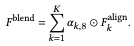### Appearance Blending

The goal of this section is to derive the appearance code $S^{blend}$ for combinatorial images. For this purpose, we introduce the LPIPS distance function used in the previous study: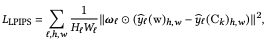$\hat{y}_l$ is the activation of the $l$-layer of convnet(VGG), $W_l, H_l$ refers to the normalized tensor in the channel dimension, and $\omega$ is the per-channel weight. In the masked case we have the following: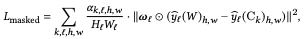A positive weight $u_k$ is obtained by learning to derive $S^{blend}$ satisfying the following equation (all $u_k$ add up to 1).

## experiment

### Experiment setup

• model
• MichiGAN
• LOHO
• Ours.
• data set
• https://arxiv.org/abs/2012.09036
• A set of 120 high-resolution images

### experimental results

User Study

We conducted a user evaluation (396 users) using Amazon's Mechanical Turk. We showed them two images of the proposed method and the existing method and asked them which one had higher image quality and fewer artifacts. 95% of them answered that the proposed method was better for LOHO and 96% of them answered that the proposed method was better for MichiGAN.

Reconstruction Reconstruction Quality

We evaluated the quality of the images by various indices, and the proposed method is the best in all indices.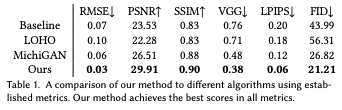Generated image

We present various combination images in the proposed method.

We have succeeded not only in combining hair (Fig. 6) but also in transferring facial features such as eyes and eyebrows (Fig. 7).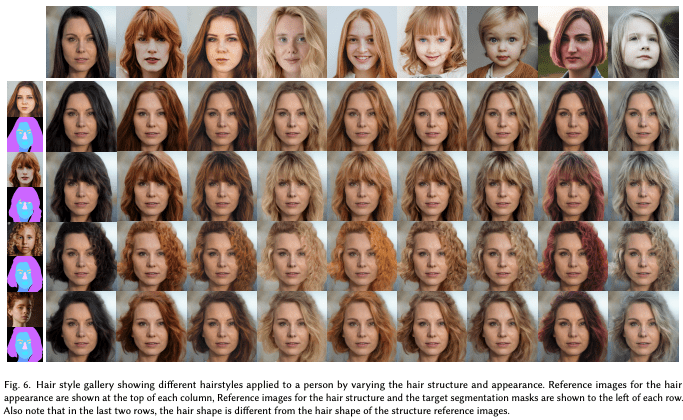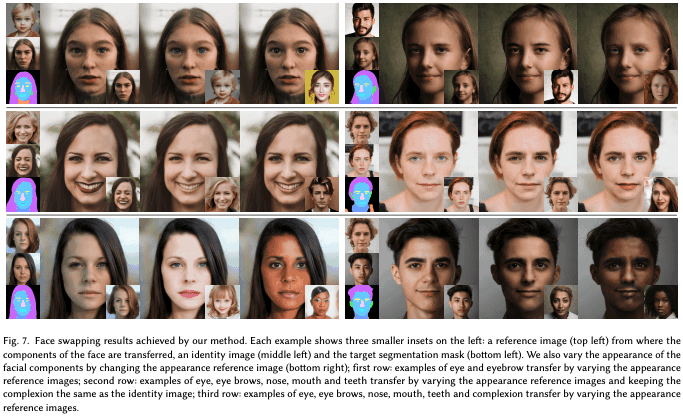・limitation

The proposed method cannot fix the geometric distortions such as the thin hair strands on the face as shown in Fig. 10. These problems may require less regularization and will be the subject of future research.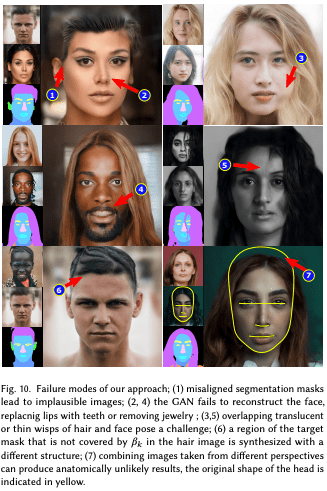## Summary

In this paper, we proposed Barbershop as a new GAN-based image editing framework. It allows users to manipulate images by manipulating segmentation masks and copying content from different images. The key features of the proposed method are, first of all, that we proposed a new latent space that combines structure tensors instead of only the commonly used W+ space. The structure tensor allows us to recognize the latent code more spatially and to capture the details of the face better. Secondly, aligned embedding allows us to embed images that are similar to the input image and to modify the image to fit the new segmentation mask. Finally, we were able to combine multiple images encoded in the new latent space to produce a high-quality image.

If you have any suggestions for improvement of the content of the article,